# Survival Regression Analysis on Customer Churn

In this post, we will analyze Telcon's Customer Churn Dataset and figure out what factors contribute to churn. By definition, a customer churns when they unsubscribe or leaves a service. With survival analysis, the customer churn event is analogous to "death". Armed with the survival function, we will calculate what is the optimum monthly rate to maximize a customers lifetime value. The source of this post and instructions to reproduce this analysis can be found at the thomasjpfan/ml-journal repo.

## Overview of the dataset¶

The dataset consist of many featuers associated with a customer. For regular survivial analysis, we only need the tenure and Churn features. The tenure is the number of time a customer has stayed with the service. The boolean Churn feature states if the customer churned or not:

df = pd.read_csv("data/WA_Fn-UseC_-Telco-Customer-Churn.csv")

Out:
tenureChurn
01No
134No
22Yes
345No
42Yes

For customers that did not churn yet, they may churn in the future. Since this is data from the future, it is not recorded in our dataset. Datasets exhibiting this behavior are called right-censored. Luckily the Cox's model is able to handle right-censored data.

We use the lifelines project to train a Cox’s Proportional Hazard model. This model is able to do regression on the other featuers in the dataset.

from lifelines import CoxPHFitter

events = convert_cat(df)
cph = CoxPHFitter()
_ = cph.fit(events, duration_col='tenure', event_col='Churn')


With the fitted survivial regression model, we take a look at how each feature affects the survivial function: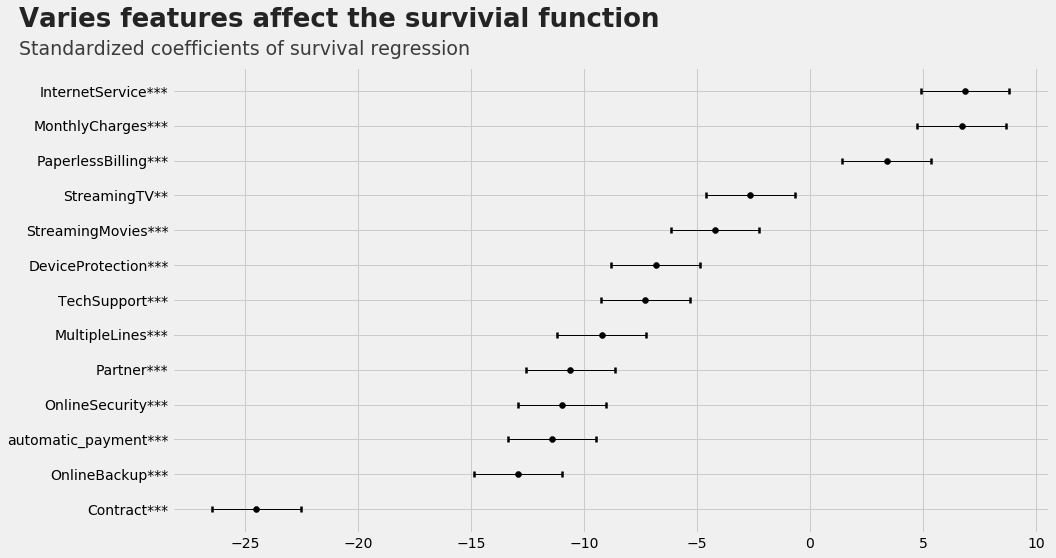The standardized cofficients gives a sense of the impact of each feature. The closer the cofficient is to zero, the less effect it has on the survivial function. The survivial function defines the probability the churn event has not occured yet at a given month, $t$: $$S(t) = P(T > t)$$ For example, when $t=0$, the probabilty $P(T > 0) = 1$, because on an infinite time scale, a customer will always churn. The boolean automatic_payment feature denotes if a customer has automatic payments enabled. We plot the survivial function with or without automatic payments: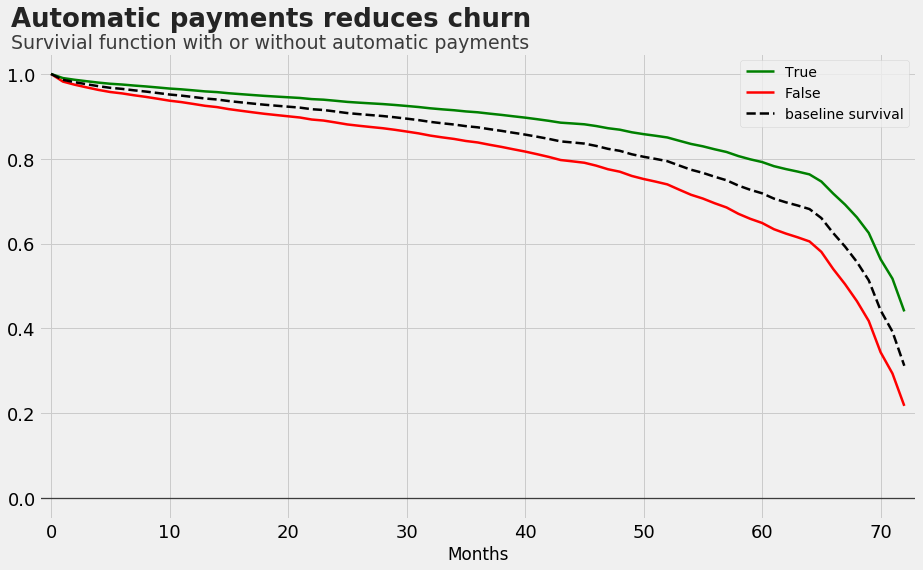The green and red curve represents the survivial function when automatic payment is on or off respectively. The result is expected, the green curve is always above the red curve, i.e. enabling automatic payments increaese the probability of survivial. The other boolean features also help with customer churn: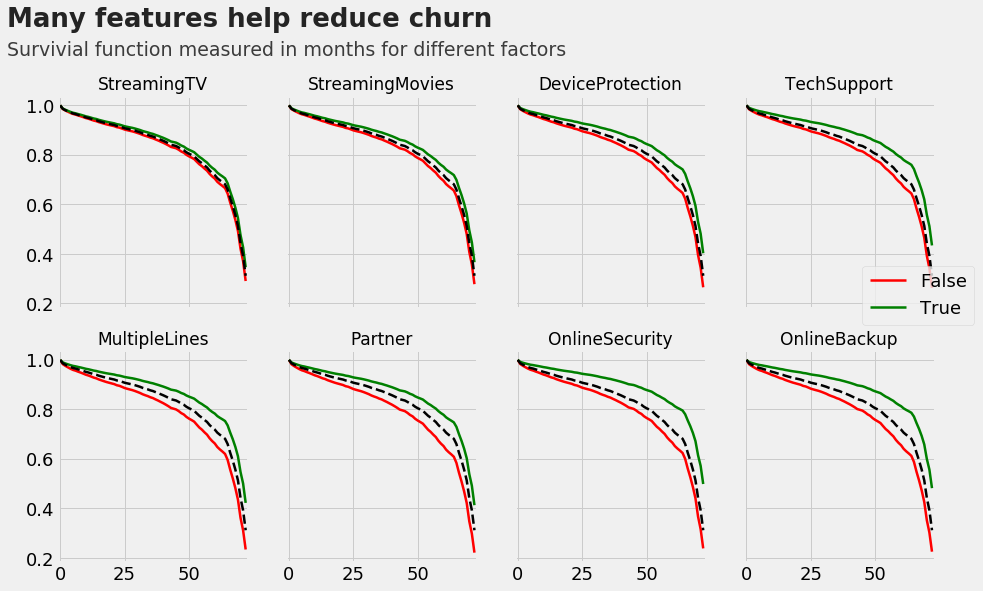The survivial function for various contract lengths shows the expected result, i.e. longer contracts prevents customers from leaving: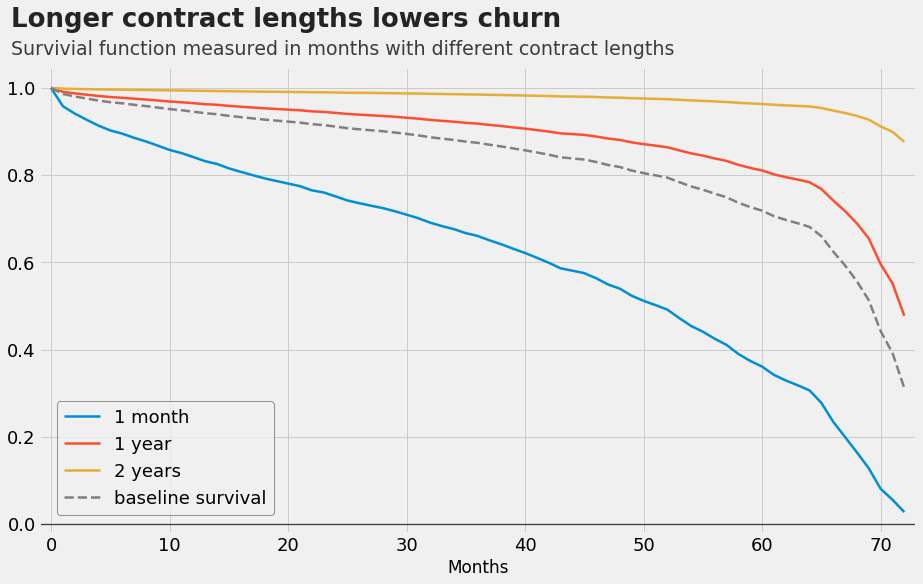## Deep dive into monthly rates¶

In this section, we will calculate how much to charge a customer to maximize lifetime value. First, we visualize the monthly rate distrubution: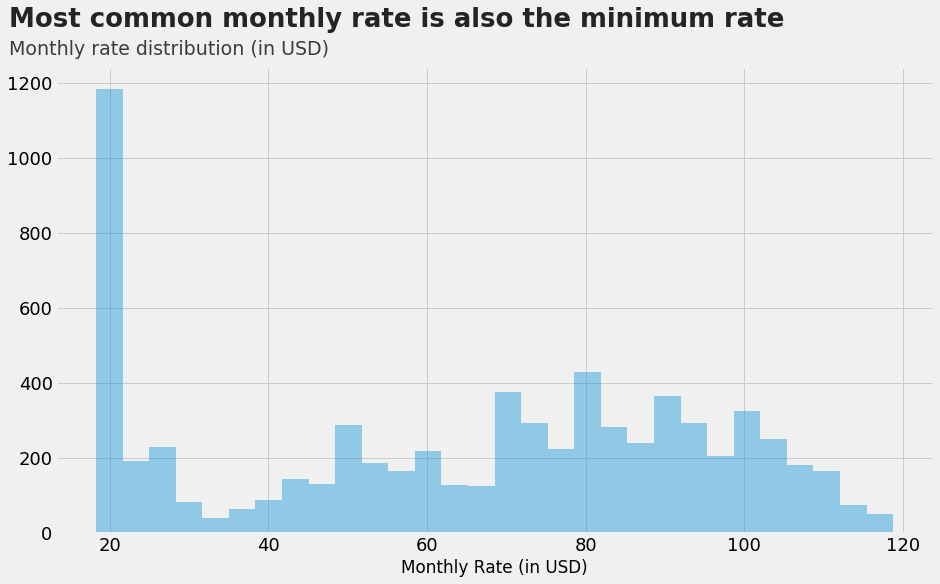Next, we plot the survivial function for different monthly rates: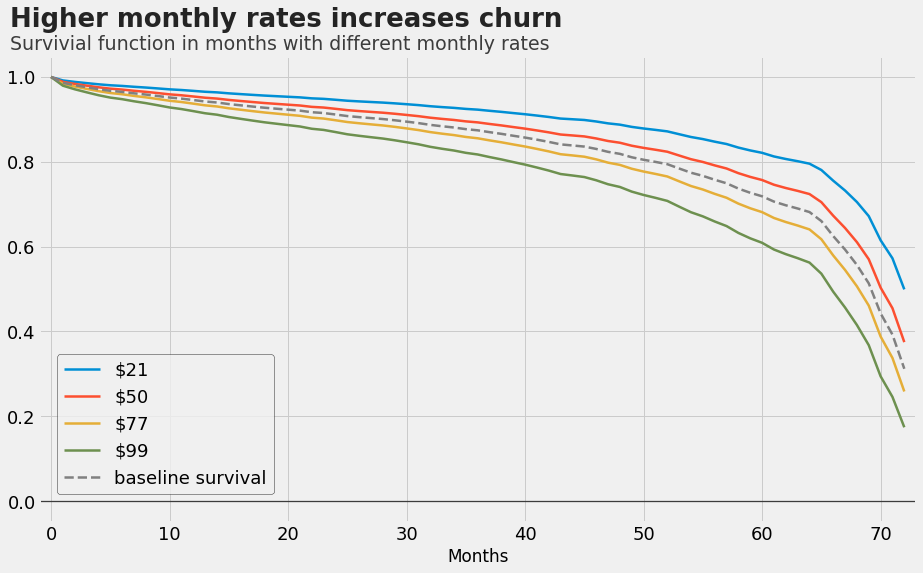Again the result is expected, the higher the monthly rate, the lower the survivial function. With these survivial functions, we can calculate the average number of months a customer will stay for different monthly rate. Multiplying the average number of months with the monthly rate, gives the lifetime value of a customer at each price point: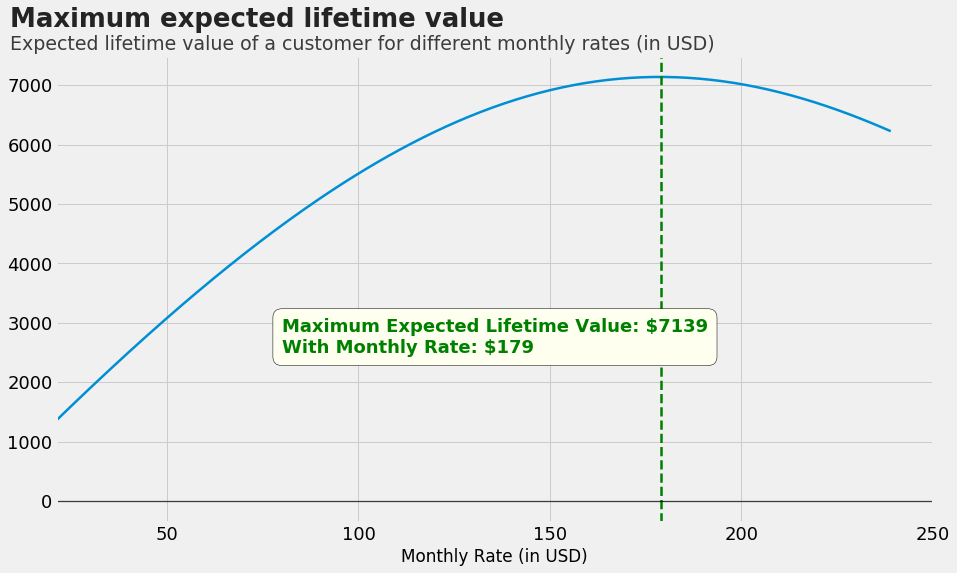In this case, the maximum expected lifetime value is 7139 USD, using a monthly rate of 179 USD.

## Whats next?¶

Survival analysis is a powerful way to look at customer churn data. We calculated the impact of each feature on the survivial curve. Moreover, we used the survival curve to calculate the expected lifetime value of a customer for various monthly rates. The next step is to do the same analysis in a bayesian point of view, which adds a measure of uncertainty into the model, enhancing our understanding of the underlying processes.

### Similar Posts

07/31/18
Nuclei Image Segmentation Tutorial
08/28/17
Rodents Of NYC
09/12/15
Bayesian Coin Flips
08/29/15
Statistical Power of Coin Flips
08/15/15
PCA Before Regression